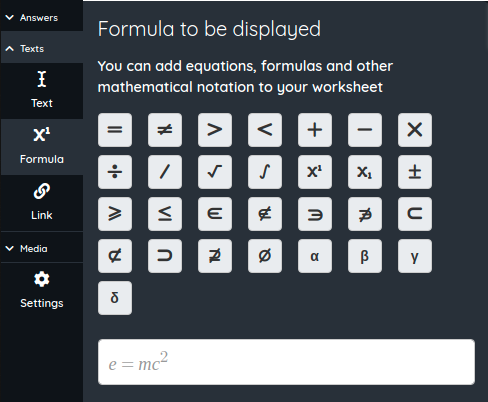# Formula

Formula field allows teachers to display mathematics notation (formulas, equations, ...) in their worksheets to better display scientific texts and questions. Always without counting as answers.To use it add a Formula field in your worksheet and input the formula or equation you want to show your students. You can use the helper buttons above to introduce symbols like square root, integral, super index, fractions and many more.

« Previous
Next »